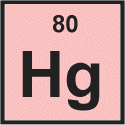# How would you find the atomic number, atomic mass, protons, neutrons and electrons for ions and isotopes? Is it similar?

Dec 22, 2013

You use the Periodic Table to get the required information.

#### Explanation:

The symbol for an isotope of an element such as $\text{O}$ is often written as ${\text{_8^16"O}}^{n}$.

• The $\text{O}$ is, of course, the symbol for an oxygen atom.

• The subscript represents the atomic number $Z$, the number of protons in the nucleus.

• The upper left superscript represents the mass number $A$, the total number of protons and neutrons in the nucleus.

• The upper right superscript represents the charge on the ion (e.g., +1 or -2).

• If there is no upper right superscript, the charge is zero, and we have a neutral atom.

How many protons, neutrons, and electrons are in an atom of mercury-201?

First, you must find the element in the Periodic Table.

All Periodic Tables give at least the symbol and the atomic number of the element.We see that for mercury the atomic number is 80.

The atomic number is the number of protons in an atom's nucleus, so we can tell right away that an atom of mercury contains 80 protons.

The mass number (201) is the total number of protons and neutrons.

So, there must be 201 - 80 = 121 neutrons.

Since atoms are electrically neutral, there must be as many electrons as there are protons.

A mercury atom needs 80 electrons to balance the 80 protons.

In summary:

• No. of protons = atomic number $Z$
• No. of electrons = atomic number $Z$
• No. of neutrons = mass number - atomic number = A – Z

How many protons, neutrons, and electrons are in ${\text{_80^200"Hg}}^{2 +}$?

Here, we have 80 protons and 200 - 80 = 120 neutrons.

The "2+" charge tells us that we have lost two electrons.

So there are 80 – 2 = 78 electrons.

Thus, the process is similar for both isotopes and ions.